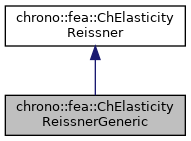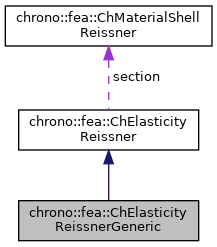chrono::fea::ChElasticityReissnerGeneric Class Reference

## Description

Generic linear elasticity for 6-field Reissner-Mindlin shells (kinematically-exact shell theory as in Witkowski et al.) to be used in a ChMaterialShellReissner.

This uses a 12x12 matrix [E] from user-input data. The [E] matrix can be computed from a preprocessing stage using a FEA analysis over a detailed 3D model of a slab of shell, hence recovering the 6x6 matrix in the linear mapping: {n,m}=[E]{e,k}.

#include <ChMaterialShellReissner.h>

Inheritance diagram for chrono::fea::ChElasticityReissnerGeneric:[legend]
Collaboration diagram for chrono::fea::ChElasticityReissnerGeneric:[legend]

## Public Member Functions

ChMatrixNM< double, 12, 12 > & Ematrix ()
Access the 12x12 [E] matrix, for getting/setting its values. More...

virtual void ComputeStress (ChVector<> &n_u, ChVector<> &n_v, ChVector<> &m_u, ChVector<> &m_v, const ChVector<> &eps_u, const ChVector<> &eps_v, const ChVector<> &kur_u, const ChVector<> &kur_v, const double z_inf, const double z_sup, const double angle) override
The FE code will evaluate this function to compute per-unit-length forces/torques given the u,v strains/curvatures. More...

virtual void ComputeStiffnessMatrix (ChMatrixRef mC, const ChVector<> &eps_u, const ChVector<> &eps_v, const ChVector<> &kur_u, const ChVector<> &kur_v, const double z_inf, const double z_sup, const double angle) override
/// Compute the 12x12 stiffness matrix [Km] , that is [ds/de], the tangent of the constitutive relation stresses/strains. More...

## Additional Inherited MembersPublic Attributes inherited from chrono::fea::ChElasticityReissner
ChMaterialShellReissnersection

## ◆ ComputeStiffnessMatrix()

 void chrono::fea::ChElasticityReissnerGeneric::ComputeStiffnessMatrix ( ChMatrixRef mC, const ChVector<> & eps_u, const ChVector<> & eps_v, const ChVector<> & kur_u, const ChVector<> & kur_v, const double z_inf, const double z_sup, const double angle )
overridevirtual

/// Compute the 12x12 stiffness matrix [Km] , that is [ds/de], the tangent of the constitutive relation stresses/strains.

Parameters
 mC tangent matrix eps_u strains along u direction eps_v strains along v direction kur_u curvature along u direction kur_v curvature along v direction z_inf layer lower z value (along thickness coord) z_sup layer upper z value (along thickness coord) angle layer angle respect to x (if needed) -not used in this, isotropic

Reimplemented from chrono::fea::ChElasticityReissner.

## ◆ ComputeStress()

 void chrono::fea::ChElasticityReissnerGeneric::ComputeStress ( ChVector<> & n_u, ChVector<> & n_v, ChVector<> & m_u, ChVector<> & m_v, const ChVector<> & eps_u, const ChVector<> & eps_v, const ChVector<> & kur_u, const ChVector<> & kur_v, const double z_inf, const double z_sup, const double angle )
overridevirtual

The FE code will evaluate this function to compute per-unit-length forces/torques given the u,v strains/curvatures.

Parameters
 n_u forces along u direction (per unit length) n_v forces along v direction (per unit length) m_u torques along u direction (per unit length) m_v torques along v direction (per unit length) eps_u strains along u direction eps_v strains along v direction kur_u curvature along u direction kur_v curvature along v direction z_inf layer lower z value (along thickness coord) z_sup layer upper z value (along thickness coord) angle layer angle respect to x (if needed) -not used in this, isotropic

Implements chrono::fea::ChElasticityReissner.

## ◆ Ematrix()

 ChMatrixNM& chrono::fea::ChElasticityReissnerGeneric::Ematrix ( )
inline

Access the 12x12 [E] matrix, for getting/setting its values.

This is the matrix that defines the linear elastic constitutive model as it maps yxz displacements "e" and xyz rotations "k" to the "n" force and "m" torque as in {n_u,n_v,m_u,m_v}=[E]{e_u,e_v,k_u,k_v}.

The documentation for this class was generated from the following files:
• /builds/uwsbel/chrono/src/chrono/fea/ChMaterialShellReissner.h
• /builds/uwsbel/chrono/src/chrono/fea/ChMaterialShellReissner.cpp